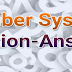## Number System formulas and Tricks for Competitive Exams

What is Number System?
Definition:
The number system or the numerical system which are representing numbers.

The number system is very important section in any type competitive exams under aptitude section. This Number system is very easy if we exercised on the various problems on the Number system questions.

Today we are sharing different type of questions are often asked in various competitive exams like Bank Clerk, Bank PO, Railways, SSC, UPSC. Aspirants suggested to exercise various types of questions and can help them in the upcoming the sarkari exams. we are providing below some different questions on number system on this article, review it once.

1 Question): On dividing a certain number by 342, we get 47 as a remainder. If the same number is divided by 18, what will be the remainder?

Solution:

Let k be the quotient,

then Number = 342 k + 47

= (18*19k)  + (18*2) + 11

hence, remainder will be 11

Note: First check whether the first Divisor (342) is divisible by new divisor (18), if that is true, then the new remainder will be the remainder from the first remainder (47) and new divisor(18).

i.e. remainder of 47/18 = 11

2 Question): What is the unit digit in the product (3547)153  x (251)72 ?

Solution:

Required digit = unit digit in 7153  x  172

= ( 74)38 x 7 x 1 = 7

3 Question): A number when successively divided by 3, 5 and 8 leaves remainder 1, 4 and 7 respectively. Find the respective remainders if the order of divisors is reversed.

Solution:

In this type of questions, we need to find the Number and then divide it with given divisors in reversed manner.

Dividend, when divisor is 8 and remainder is 7 = 8 x 1 + 7 = 15

Dividend, when divisor is 5 and remainder is 4 = 5 x 15 + 4 = 79

Dividend, when divisor is 3 and remainder is 1 = 3 x 79 + 1 = 238

Hence, Number or Dividend is 238, and we divide it with 8, 5 and 3 (normal division) it will leave the remainders 6,4 and 2 respectively.

4 Question ): On dividing a number by 5, we get 3 as a remainder. what will be the remainder when the square of this number is divided by 5?

Solution:

remainder = remainder of square of 3 divided by 5 = 4

5 Question): On dividing 2272 as well as 875 by 3 digit number N, we get the same remainder. The sum of the digits of N is:

Solution:

Here (2272 - 875)= 1397 is also exactly divisible by N,

1397 = 11x127 => required three digit number is 127, whose digit sum = 10

6 Question): What will be the remainder when (6767 + 67) is divided by 68?

Solution:

(xn + 1) will be divisible by (x+1) when x is odd.

Therefore,  (6767 + 1) will be divisible by (67 +1)

or,  (6767 + 1) + 66 when divided by 68 will give remainder 66

7 Question): Which one of the following numbers will completely divide (461 + 462 + 463 + 464)?

Solution: 461 ( 1 + 4 + 16 + 64 ) = > 461 x 85 =>  460 x 360 => which is divisible by 10.

8 Question): When a number is divided by 13, the remainder is 11. When the same number is divided by 17, the remainder is 9. What is the number?

Solution:

Number = 13p+11 = 17q+9 => q= (2+13p)/17

the least value of p for which q is whole number is 26.

hence, number = (13 x 26 + 11) = 349

9 Question): The sum of how many terms of the series 6 + 12 + 18 + 24 + ... 1800 ?

Solution: here, a = 6; d=6,

we know, Sn = n/2 [ 2a + (n-1)d ]  => 1800 = n/2[ 12 +(n-1)6 ] => n=24

10 Question): (22 + 42 + 62 +…+202)

Solution:

22(12 + 22 + 32 +…+102) => 4 (1/6 * 10 * 11 * 21) = 1540

Question 11): Find the sum of all the odd numbers from 1 to 101.

Solution:

The required Sum = Sum of all the odd numbers from 1 to 100 - Sum of all the odd numbers from 1 to 20.

= Sum of first 51 odd numbers -  Sum of first 10 odd numbers

= 502 - 202 = 2501

Question 12): Find the greatest number of 5 digits which is exactly divisible by 137.

Solution:

The greatest number of 5 digits is 99999, on dividing it by 137 we get 126 as a remainder.

therefore the required number = 99999 - 137 = 99873

Question 13): The Sum of two numbers is 14 and their difference is 10. Find the product of the two numbers.

Solution:

(x+y)2 – (x-y)2 = 4xy

142 -102 = 4xy

xy = 24

Question 14): The sum of two digit numbers is 8. If the digits are reversed, the number is decreased by 54. Find the number.

Solution:

x-y = dec. in number /9 = 54/9 = 6

x+y = 8

solving both, we get, x=7 and y=1.

Question 15): How many numbers between 100 and 300 are divisible by 7?

Solution:

On diving the difference (300 - 200) by 7 we get 28 as quotient and 4 as a remainder, Hence, there are 28 such numbers.

P.S. : This Set of questions is prepared For Last Minutes Revision for ssc cgl, chsl, fci , ibps bank po and other exams.

Number System formulas and Tricks for Competitive ExamsReviewed by SSC NOTES on August 21, 2023 Rating: 5